Courses

# Capacitance Physics Notes | EduRev

## Physics : Capacitance Physics Notes | EduRev

The document Capacitance Physics Notes | EduRev is a part of the Physics Course Basic Physics for IIT JAM.
All you need of Physics at this link: Physics

Introduction:

A useful device for storing electrical energy consists of two conductors in close proximity and insulated from each other. A simple example of such a storage device is the parallel-plate capacitor. If positive charges with total charge +Q are deposited on one of the conductors and an equal amount of negative charge −Q is deposited on the second conductor, the capacitor is said to have a charge Q. As shown in Figure 11, it consists of two flat conducting plates, each of area A, parallel to each other and separated by a distance d.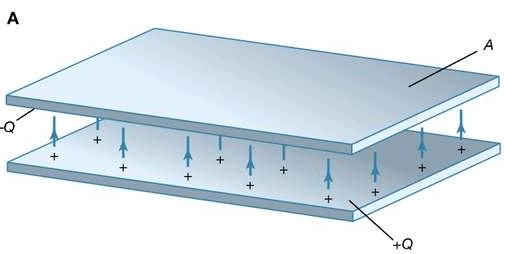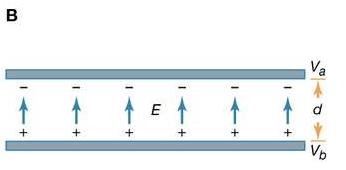Figure 11: Parallel-plate capacitor. (A) This storage device consists of two flat conducting plates, each of area A. (B) These plates are parallel and separated by a small distance d.

Principle of the Capacitor:

To understand how a charged capacitor stores energy, consider the following charging process. With both plates of the capacitor initially uncharged, a small amount of negative charge is removed from the lower plate and placed on the upper plate. Thus, little work is required to make the lower plate slightly positive and the upper plate slightly negative. As the process is repeated, however, it becomes increasingly difficult to transport the same amount of negative charge, since the charge is being moved toward a plate that is already negatively charged and away from a plate that is positively charged. The negative charge on the upper plate repels the negative charge moving toward it, and the positive charge on the lower plate exerts an attractive force on the negative charge being moved away. Therefore, work has to be done to charge the capacitor.
Where and how is this energy stored? The negative charges on the upper plate are attracted toward the positive charges on the lower plate and could do work if they could leave the plate. Because they cannot leave the plate, however, the energy is stored. A mechanical analogy is the potential energy of a stretched spring. Another way to understand the energy stored in a capacitor is to compare an uncharged capacitor with a charged capacitor. In the uncharged capacitor, there is no electric field between the plates; in the charged capacitor, because of the positive and negative charges on the inside surfaces of the plates, there is an electric field between the plates with the field lines pointing from the positively charged plate to the negatively charged one. The energy stored is the energy that was required to establish the field. In the simple geometry of Figure 11, it is apparent that there is a nearly uniform electric field between the plates; the field becomes more uniform as the distance between the plates decreases and the area of the plates increases. It was explained above how the magnitude of the electric field can be obtained from the electric potential. In summary, the electric field is the change in the potential across a small distance in a direction perpendicular to an equipotential surface divided by that small distance. In Figure 11, the upper plate is assumed to be at a potential of Va volts, and the lower plate at a potential of Vb volts. The size of the electric field is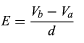(10)
in volts per metre, where d is the separation of the plates. If the charged capacitor has a total charge of +Q on the inside surface of the lower plate (it is on the inside surface because it is attracted to the negative charges on the upper plate), the positive charge will be uniformly distributed on the surface with the value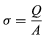(11)
in coulombs per metre squared. Equation (8) gives the electric field when the surface charge density is known as E = σ/ε0. This, in turn, relates the potential difference to the charge on the capacitor and the geometry of the plates. The result is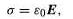(8)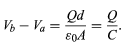(12)

The quantity C is termed capacity; for the parallel-plate capacitor, C is equal to ε0A/d. The unit used for capacity is the farad (F); one farad equals one coulomb per volt. In equation (12), only the potential difference is involved. The potential of either plate can be set arbitrarily without altering the electric field between the plates. Often one of the plates is grounded—i.e., its potential is set at the Earth potential, which is referred to as zero volts. The potential difference is then denoted as ΔV, or simply as V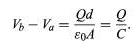(12)

Three equivalent formulas for the total energy W of a capacitor with charge Q and potential difference V are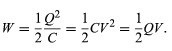(13)

All are expressed in joules. The stored energy in the parallel-plate capacitor also can be expressed in terms of the electric field; it is, in joules,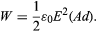(14)
The quantity Ad, the area of each plate times the separation of the two plates, is the volume between the plates. Thus, the energy per unit volume (i.e., the energy density of the electric field) is given by 1/2ε0E2 in units of joules per metre cubed.

Applications Of Capacitors:

Capacitors have many important applications. They are used, for example, in digital circuits so that information stored in large computer memories is not lost during a momentary electric power failure; the electric energy stored in such capacitors maintains the information during the temporary loss of power. Capacitors play an even more important role as filters to divert spurious electric signals and thereby prevent damage to sensitive components and circuits caused by electric surges.

Offer running on EduRev: Apply code STAYHOME200 to get INR 200 off on our premium plan EduRev Infinity!

,

,

,

,

,

,

,

,

,

,

,

,

,

,

,

,

,

,

,

,

,

;